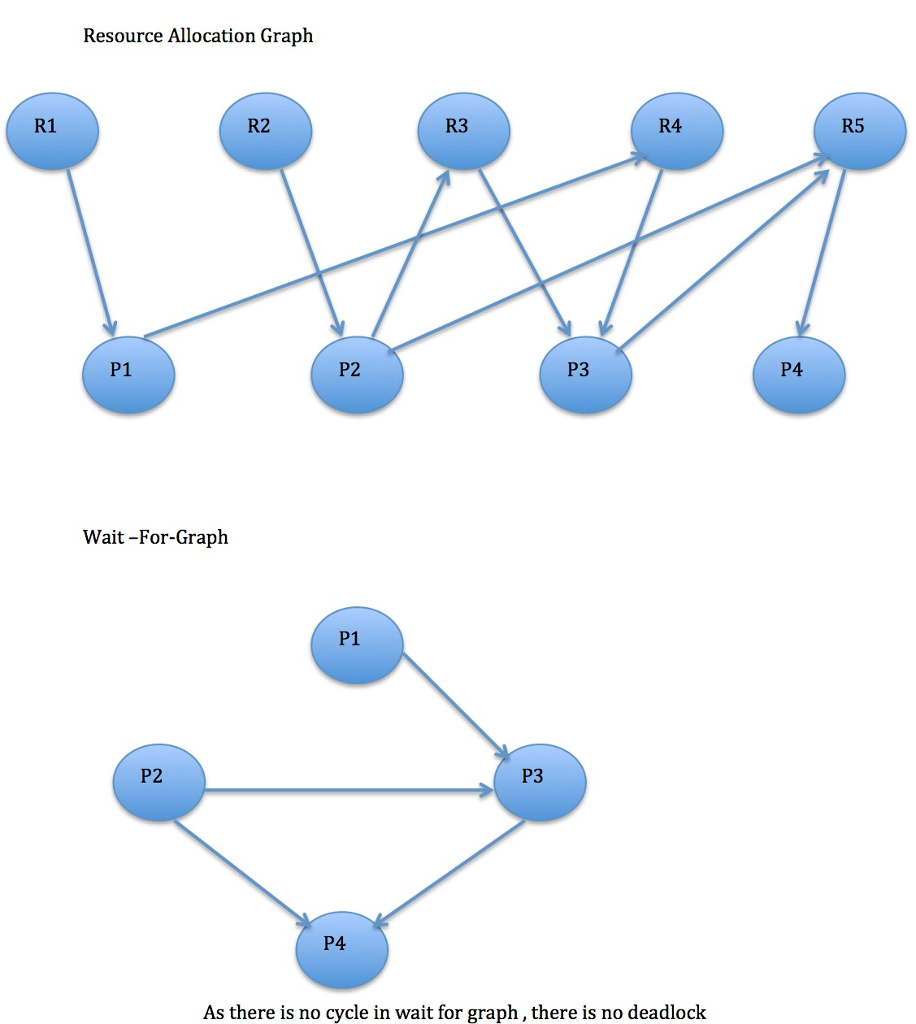# Answered! You are given a system of 4 processes (P1-P4) and 5 resource types R1-R5, one instance of each. The current state is…

You are given a system of 4 processes (P1-P4) and 5 resource types R1-R5, one instance of each. The current state is as follows: P1 is holding R1 and waiting on R4. P2 is holding R2 and waiting on R3 and R5. P3 is holding R3 and R4, and waiting on R5. P4 is holding R5. Draw a resource allocation graph and a wait-for-graph. From WFG, determine if there is a deadlock? Explain your answer.